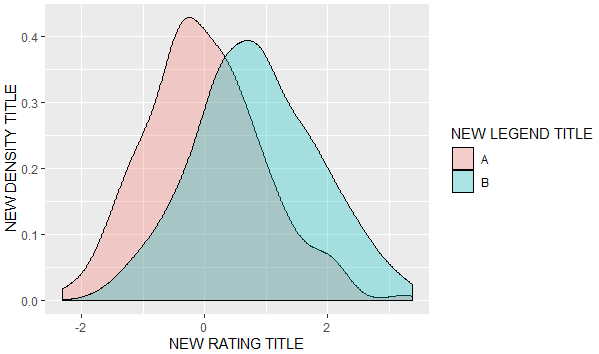2 views

I have the following plot like below. It was created with this command:

library(ggplot2)

df <- data.frame(cond = factor(rep(c("A", "B"), each = 200)),

rating = c(rnorm(200), rnorm(200, mean=.8)))

ggplot(df, aes(x=rating, fill=cond)) +

geom_density(alpha = .3) +

xlab("NEW RATING TITLE") +

ylab("NEW DENSITY TITLE")

Now next thing I want to do is to modify the legend title from cond into NEW LEGEND TITLE.

So what I did is to just add the following line add the end of the above code:

+labs(colour="NEW LEGEND TITLE")

But it doesn't work. What's the right way to do it?by

To change the legend title of your plot, add the following layer:

labs(fill = "New Legend Title")

i.e.,

ggplot(df, aes(x=rating, fill=cond)) +

geom_density(alpha = .3) +

xlab("NEW RATING TITLE") +

ylab("NEW DENSITY TITLE")+

labs(fill = "NEW LEGEND TITLE")

Output:by (32.3k points)

I think your approach isn't working because you have used fill=cond in ggplot():

+ labs(color='NEW LEGEND TITLE')

However, if you replace color by fill, it will work finely:

+ labs(fill='NEW LEGEND TITLE')